#2018 iT 邦幫忙鐵人賽DAY 21
0
Modern Web

source code

• 棋盤
• 小溪
• 潛動

# 1. 棋盤

## 1.1 條紋

``````heightmapValue.x = pow(sin(uv.x * 200.0), 0.5) * 10.0;
``````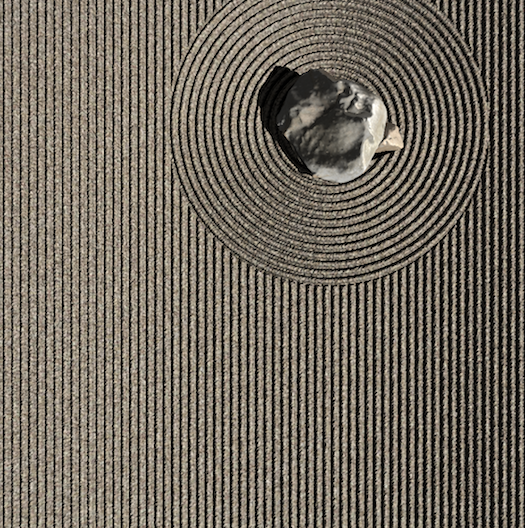## 1.2 Grid

``````float unit = 1.0 / floor(uGridUnit);

// gridX, gridY 若為 0，即代表偶數格。為 1，則是奇數格。
float gridX = mod(uv.x / unit, 2.0);
float gridY = mod(uv.y / unit, 2.0);

if ((gridX < 1.0 && gridY < 1.0) || (gridX >= 1.0 && gridY >= 1.0)) {
heightmapValue.x = pow(sin(uv.y * 200.0), 0.5) * 10.0 * uBackgroundWaveScale;
}
``````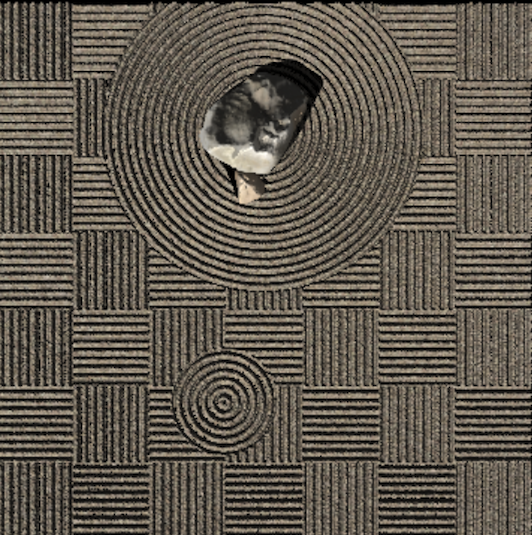# 2. 小溪

``````float angle = uv.x * 200.0;
float wave = sin(uv.y * 10.0) * 8.0;
float sum = angle + wave;
// heightmapValue.x 即為高度
heightmapValue.x = pow(sin(sum), 0.5) * 10.0 * uBackgroundWaveScale;
``````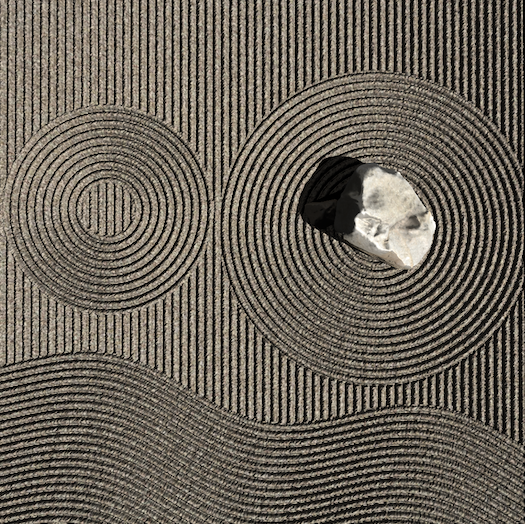## 2.1 旋轉矩陣

``````mat2 uWaveTransform = mat2(0.8, 0.6, -0.6, 0.8);
vec2 uvNew = uWaveTransform * uv;
//而後面原本是用 uv 的改成使用 uvNew 就可以產生旋轉效果了！
``````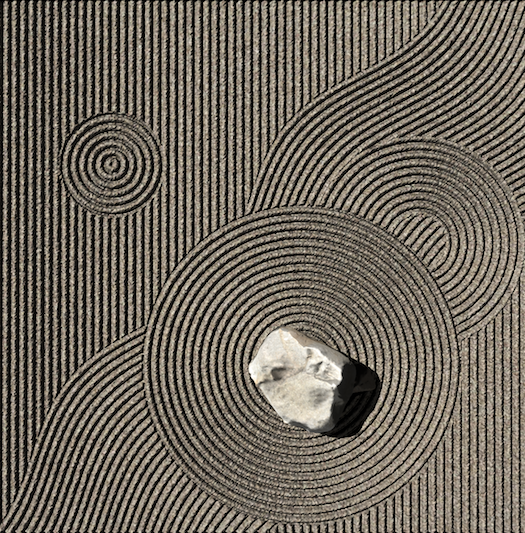## 2.2 數字實驗室

``````float angle = uv.x * uv.y * 200.0;
float wave = sin(uvNew.y * 10.0 + sin(uvNew.x * 10.0) * 0.8) * 8.0;
``````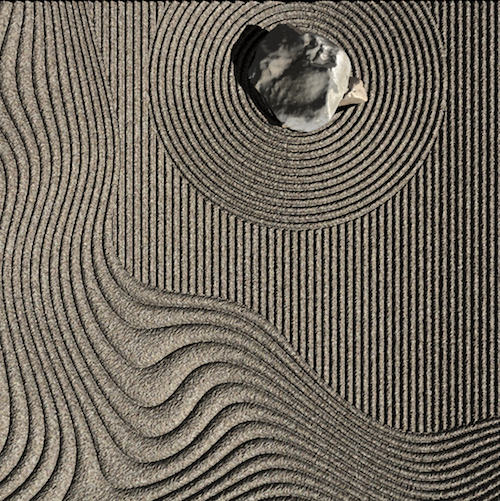``````float angle = uv.x * uv.y * 200.0;
float wave = sin(uvNew.y * 10.0 + sin(uvNew.x * 10.0) * 0.8) * 8.0;
``````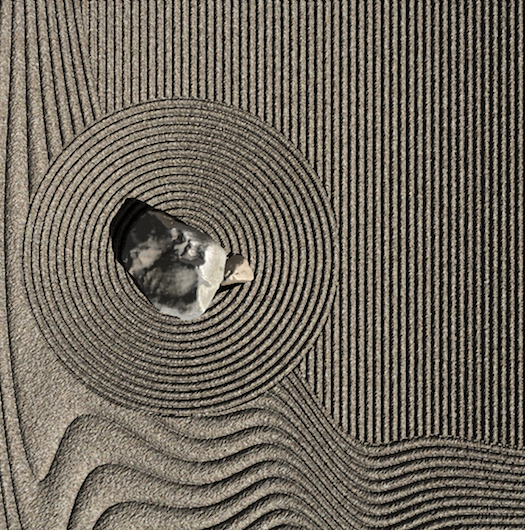``````float angle = uv.x * uv.y * 200.0;
float wave = sin(uvNew.y * 10.0 + sin(uv.y * 10.0) * 2.0) * 8.0;
``````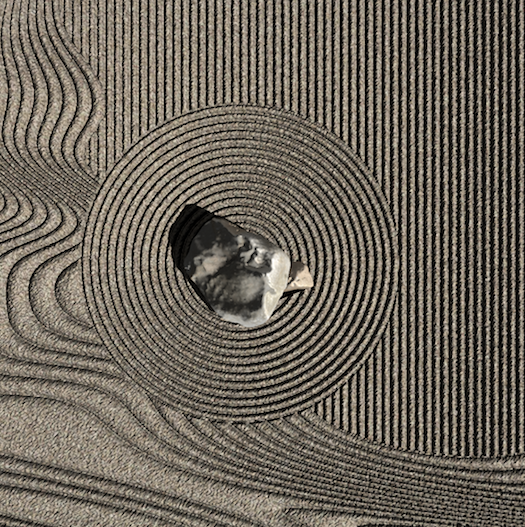# 3. 潛動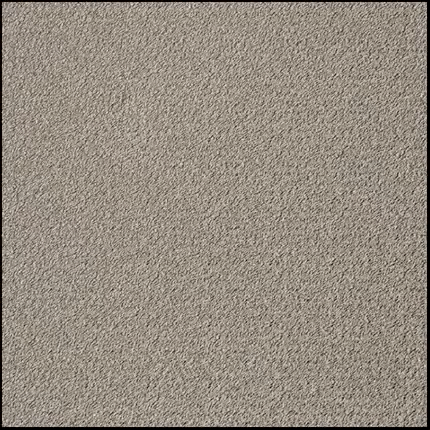``````// uCircularWave.xy 代表的是石頭的位置
float d = length(uv - uCircularWave.xy);

// uMasterScale 會在一段時間裡面從 0 逐漸變化到 1，或是 1 到 0
heightmapValue.x *= pow(uMasterScale, (d + 0.02) * 15.0);
``````